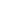Flat 50% off

Ends in

###### Square root of a Complex Number, CBSE 11th Mathematics in English
2,246 views###### Square root of a Complex Number, CBSE 11th Mathematics in English

Published on 30, Jul 2019

This video lecture is about Square root of a Complex Number from chapter Complex Number of Mathematics, online video lecture for CBSE class 11th students, prepared by the best-experienced faculty of Misostudy (https://www.misostudy.com). Any nonzero complex number also has two square roots. For example, using the imaginary unit I, the two square roots of are . The principal square root of a number is denoted (as in the positive real case) and is returned by the Wolfram Language function Sqrt[z] In this online video lecture, we will discuss the Theory and Problem Solving of Complex Number from Mathematics for the preparation of CBSE 2020 exam. Misostudy's courses will help students to boost CBSE preparation to perform best in exams and achieve a good rank.